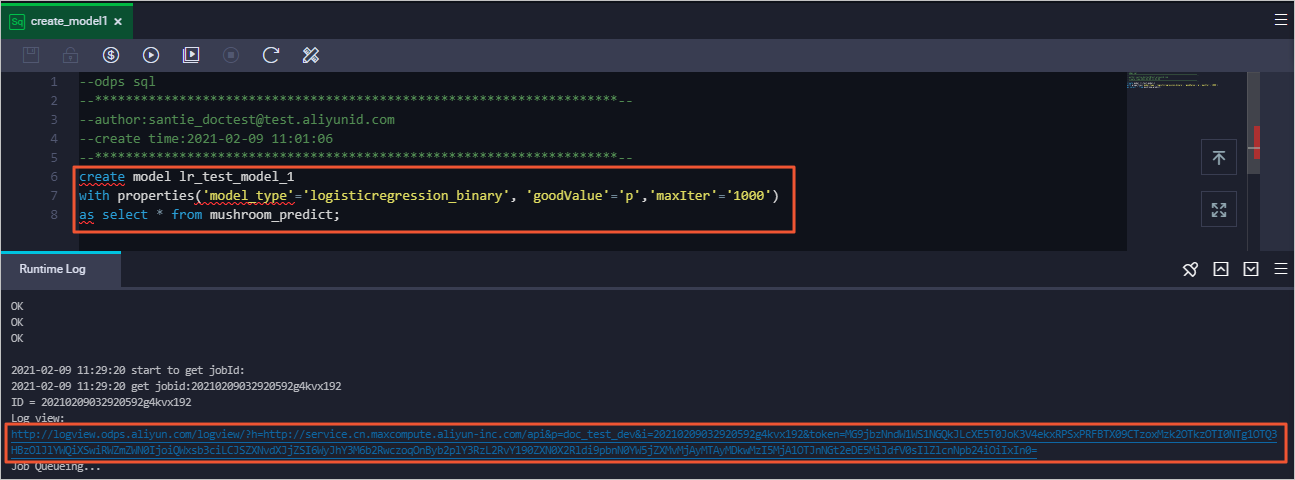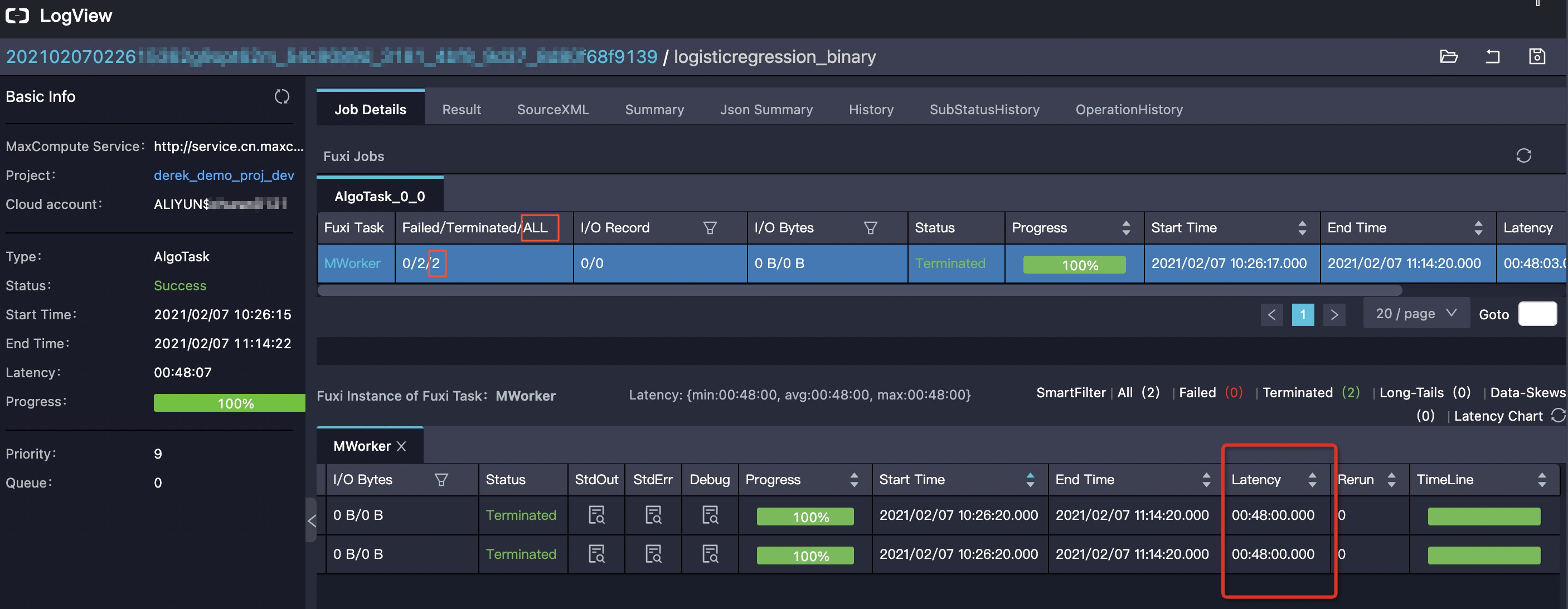This topic describes how to estimate the cost of a MaxCompute SQLML job.

## Background information

In most cases, each algorithm component consists of multiple subtasks. To calculate the cost, you must first calculate the costs of the subtasks in each algorithm component and sum up the costs of all the components. MaxCompute and Machine Learning Platform for AI (PAI) are used to run a MaxCompute SQLML job. Therefore, the cost of the MaxCompute SQLML job consists of the costs generated for both MaxCompute and PAI.

## Billing rules

The underlying machine learning capabilities of MaxCompute SQLML are independently charged based on the billing rules for PAI. Therefore, the cost of a MaxCompute SQLML job is calculated based on the following parts:
• SQL job: The computing and storage costs of an SQL job are calculated based on the billing rules for MaxCompute SQL. For more information, see Billing method.
• Machine learning model: The cost of a machine learning model is calculated based on the billing rules for Machine Learning Studio. The following table describes the rules. For more information about PAI billing, see Billing of Machine Learning Studio.
Machine learning model Unit price (CNY per billable hour) Region
Logistic regression for binary classification 1.3
• China (Beijing)
• China (Shanghai)
• China (Hangzhou)
• China (Shenzhen)
• China (Hong Kong)
Logistic regression for multiclass classification
Linear regression
Note One billable hour is equivalent to the usage of one CPU core and 4 GB of memory for one hour. The number of billable hours is calculated by using the following formula:
``Number of billable hours = Max(Number of CPU cores × Duration,Memory size × Duration/4)``
The following formula shows how to calculate the number of billable hours if you use 2 CPU cores and 5 GB of memory for one hour:
``Number of billable hours = Max(2 × 1,5 × 1/4) = 2``
The bill is calculated by using the following formula:
``````Bill = Number of billable hours × Unit price
In this example, the bill is calculated by using the following formula:
2 (Billable hours) x 1.3 (Unit price) = CNY 2.6``````

## Billing example

You must obtain the usage of CPU cores and memory to estimate the cost based on the billing rules for MaxCompute SQLML.

In this example, an existing prediction dataset in Quick start is used to estimate the cost.

1. Use the ad hoc query feature of DataWorks, create a logistic regression for binary classification model based on the prediction dataset mushroom_predict, and then obtain the Logview URL. The model is named lr_test_model_1.
Sample statement:
``````create model lr_test_model_1
with properties('model_type'='logisticregression_binary', 'goodValue'='p','maxIter'='1000')
as select * from mushroom_predict;``````
The following results are returned.2. Log on to Logview based on the Logview URL and obtain the instance ID of the AlgoTask job in Logview.
A PAI job is defined as an AlgoTask job in MaxCompute. The naming convention for an AlgoTask job is `Projectname+'_'+InstanceID+"AlgoTask_x_x"`. You can obtain the instance ID from the job name.3. Use the ad hoc query feature of DataWorks and obtain the Logview URL of the PAI job based on the instance ID.
Sample statement:
``wait 20210209031144617gcjpt62m_1dbea3a8_17de_40d5_80bf_ce19e11d****;``
The following results are returned.4. Log on to Logview based on the Logview URL of the PAI job. Go to the SourceXML tab and query the usage of CPU cores and memory for TaskPlan.In the preceding rules:
• The number of used CPU cores is calculated by dividing the value of CPU by 100. In this example, one CPU core is used to run the job.
• The unit of Memory is MB. In this example, 3315 MB (3.2 GB) of memory is used to run the job.
Notice The preceding results show the CPU cores and memory that are used by a subtask. A job may have multiple subtasks. To calculate the number of subtasks for a job, perform the next step.
5. Log on to Logview based on the Logview URL of the PAI job and obtain the number and duration of subtasks on the Job Details tab.
The following figure shows that two subtasks exist and the duration for each subtask is 48 minutes.In this example, the number of used CPU cores is 2 (2 x 1), the used memory size is 6.4 GB (2 x 3.2 GB), and the duration is 48 minutes.

6. Estimate the total cost.
1. Calculate the number of billable hours.
``Number of billable hours = Max(Number of CPU cores x Duration,Used memory size x Duration/4) = Max[2 x 1x (48 x 60/3600),2 × 3.2 × (48 x 60/3600)/4] ≈ 1.6``
2. Calculate the cost of the PAI job.
``Cost of a PAI job = Number of billable hours for subtasks × Unit price ≈ 1.6 × 1.3 = CNY 2.08``
The cost of the PAI job in this example is CNY 2.08.
3. Calculate the cost of the SQL job. For more information, see Computing pricing.
4. The sum of the costs for the PAI job and the SQL job equals the cost of a MaxCompute SQLML job.